General "Transformation" Vocabulary MathBitsNotebook.com Terms of Use   Contact Person: Donna RobertsThese are general terms that you will see in your study of transformations.
Remember that transformations are operations that alter the form of a figure.
The standard transformations are reflections, translations, rotations, and dilations.

Terms are listed in alphabetical order.

 Image: An image is the resulting point or set of points under a transformation.  For example, if the reflection of point P in line l is P' (referred to as P prime), then P' is called the image of point P under the reflection (or the pre-image).  Such a transformation is denoted rl (P) = P'.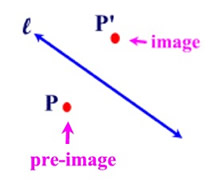Invariant: A figure or property that remains unchanged under a transformation of the plane is referred to as invariant (not varying).  No variations have occurred.

 Opposite Transformation: An opposite transformation is a transformation that changes the orientation of a figure.  Reflections and glide reflections are opposite transformations.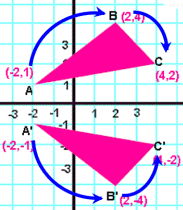For example, the original image, triangle ABC, has a clockwise orientation - the letters A, B and C are read in a clockwise direction.  After the reflection in the x-axis, the image triangle A'B'C' has a counterclockwise orientation - the letters A', B', and C' are read in a counterclockwise direction. A reflection is an opposite transformation.

 Orientation: Orientation refers to the arrangement of points, relative to one another, after a transformation has occurred.  For example, the reference made to the direction traversed (clockwise or counterclockwise) when traveling around a geometric figure.  (Also see the diagram shown under "Opposite Transformations".)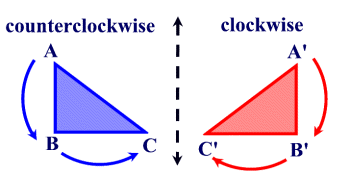Rigid Transformation (or Isometry): A rigid transformation, or isomentry, is a transformation of the plane that preserves length. For example, if the sides of an original pre-image triangle measure 3, 4, and 5, and the sides of its image after a transformation measure 3, 4, and 5, the transformation preserved length. A direct isometry preserves orientation or order - the letters on the diagram go in the same clockwise or counterclockwise direction on the figure and its image.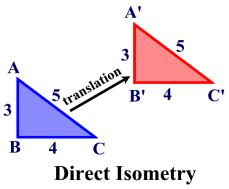A non-direct or opposite isometry changes the order (such as clockwise changes to counterclockwise).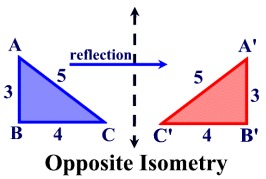Transformation: A transformation of the plane is a one-to-one mapping (or moving) of points in the plane to points in the plane. In the plane, a mapping will carry ordered pairs to new locations according to some specified rule.

 Transformational Geometry: Transformational Geometry is a method for studying geometry that illustrates congruence and similarity by the use of transformations.

 Transformational Proof: A transformational proof is a proof that employs the use of transformations to arrive at its conclusion.